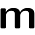This blog has moved to www.mscroggs.co.uk.

## Sunday, 13 April 2014

### Sunday Afternoon Maths VIII

Here's this week's collection. Answers & extensions here. Why not discuss the problems on Twitter using #SundayAfternoonMaths or on Reddit.

#### Two

Find a such that a+(a+A)-1 = 2, where A = (a+A)-1.
ie. a + /(a+1/(a+1/(a+1/(a+1/(a+1/(a+...)))))) = 2.

Find b such that b+(b+B)0.5 = 2, where B = (b+B)0.5.
ie. b + √(b+√(b+√(b+√(b+√(b+√(b+...)))))) = 2.

Find c such that c+(c+C)2 = 2, where C = (c+C)2.

In terms of k, find d such that d+(d+D)k = 2, where D = (d+D)k.

#### Reverse Bases Again

Find three digits a, b and c such that abc in base 10 is equal to cba in base 9?

#### Adding bases

Let ab denote a in base b.
Find bases A, B and C less than 10 such that 12A+34B=56C

#### Complex Squares

For which complex numbers, z, are Re(z2) and Im(z2) both positive?

#### Rebounds

In a 4x3 rectangle, a ball is fired from the top left corner at 45°.It bounces around a rectangle until it hits a corner. Which corner does it end in?
Which corner will it end in for rectangles of other sizes?

#### 2 comments:

1.Did you mean something slightly different for complex squares? You've written Re(z^2) twice.

1.Yes I meant Re and Im. It's changed now